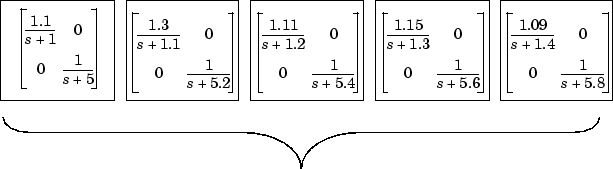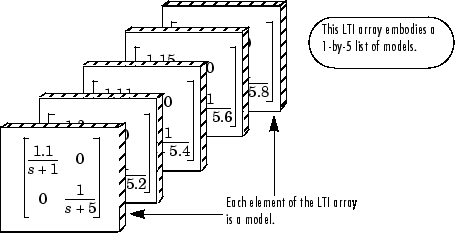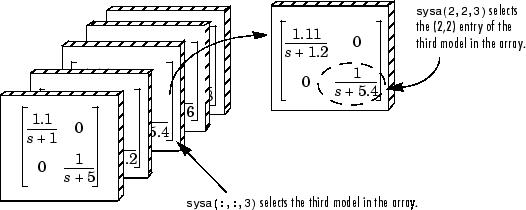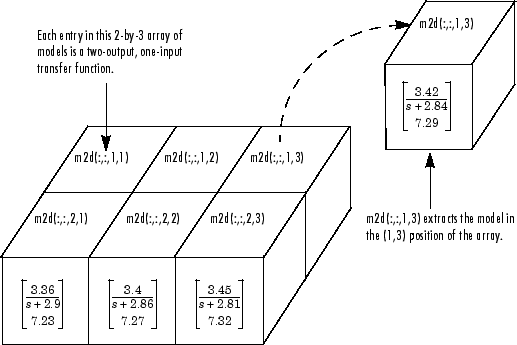Model Arrays

What Are Model Arrays?

In many applications, it is useful to consider collections of multiple model objects. For example, you may want to consider a model with a parameter that varies across a range of values, such as

sys1 = tf(1, [1 1 1]);
sys2 = tf(1, [1 1 2]);
sys3 = tf(1, [1 1 3]);

and so on. Model arrays are a convenient way to store and analyze such a collection. Model arrays are collections of multiple linear models, stored as elements in a single MATLAB® array.

For all models collected in a single model array, the following attributes must be the same:

• The number of inputs and outputs

• The sample time Ts

• The time unit TimeUnit

Uses of Model Arrays

Uses of model arrays include:

• Representing and analyzing sensitivity to parameter variations

• Validating a controller design against several plant models

• Representing linear models arising from the linearization of a nonlinear system at several operating points

• Storing models obtained from several system identification experiments applied to one plant

Using model arrays, you can apply almost all of the basic model operations that work on single model objects to entire sets of models at once. Functions operate on arrays model by model, allowing you to manipulate an entire collection of models in a vectorized fashion. You can also use analysis functions such as bode, nyquist, and step to model arrays to analyze multiple models simultaneously. You can access the individual models in the collection through MATLAB array indexing.

Visualizing Model Arrays

To visualize the concept of a model array, consider the set of five transfer function models shown below. In this example, each model has two inputs and two outputs. They differ by parameter variations in the individual model components.Just as you might collect a set of two-by-two matrices in a multidimensional array, you can collect this set of five transfer function models as a list in a model array under one variable name, say, sys. Each element of the model array is a single model object.

Visualizing Selection of Models From Model Arrays

The following illustration shows how indexing selects models from a one-dimensional model array. The illustration shows a 1-by-5 array sysa of 2-input, 2-output transfer functions.The following illustration shows selection of models from the two-dimensional model array m2d.